Egwald Mathematics: Linear Algebra

Vectors

by

Elmer G. Wiens

Egwald's popular web pages are provided without cost to users.
Please show your support by joining Egwald Web Services as a Facebook Fan:Follow Elmer Wiens on Twitter:Definition of a Vector.

A vector is a mathematical object with magnitude and direction used to represent items such as the velocity, acceleration, or momentum of an object. A vector v can be represented by an n-tuple of real numbers:

v = [vi] = (v1, v2, . . . . . , vn)

considered to be elements (points) of Rn, an n-dimensional real space.

If the n-tuples are complex numbers, then v is an element of Cn, an n-dimensional complex space.

The 2-dimensional vector v = (v1, v2) in the diagram below has magnitude 12.81, the distance from the origin to (8, 10), and direction, the orientation of the arrow from the origin to (8, 10). Notice that the v1 component is measured along the x-axis, while the v2 component is measured along the y-axis.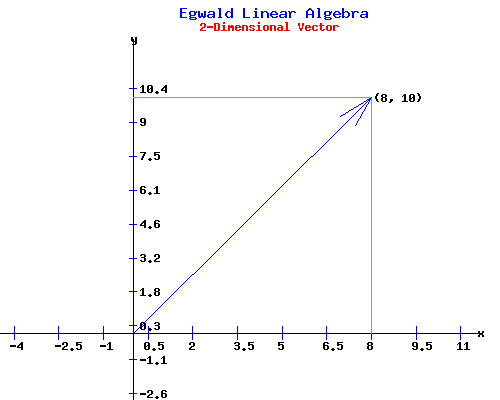Diagram a Vector v = [v1, v2]
v1: v2:

You can change the vector in the form above to see how its diagram changes. For example, try v = (-22, 15) and click "Vector".

The 3-dimensional vector a = (a1, a2, a3) in the diagram below has magnitude 17.83, the distance from the origin to (13, 10, 7), and direction, the orientation of the line from the origin to (13, 10, 7). Notice that the a1 component is measured along the x-axis, the a2 component is measured along the y-axis, and the a3 component is measured along the z-axis.For example, the acceleration of an object at 17.83 meters per second2 in the (13, 10, 7) direction can be represented by the line in the preceding diagram.

Rules of Operations on Vectors.

Scalar Multiplication of a Vector.

The product of a vector v = [vi] in Rn by a number s is [s*vi]. A real number scalar is an element of R1 = R.

To multiply a scalar by a vector, simply multiply each component of the vector by the scalar.

For example, the linear momentum p of an object is the product of its velocity, a vector v, and its mass, a scalar m:

p = m * v.

Addition of Vectors.

Given vectors v = [vi] and w = [wi] in Rn, their sum v + w is another vector u = [ui] in Rn, where:

ui = vi + wi  , for i = 1, . . . n .

To add two vectors, simply add the two vectors components.

Subtraction of Vectors.

Given vectors v and w of dimension n, their difference w - v is another vector u of dimension n, where:

u = w - v = w + (-1) * v.

The next diagram shows v + w in red, and w - v in green, where v = (6, 3), and w = (2, 7).Adding and Subtracting
v = [v1, v2] & w = [w1, w2]
v1: v2:
w1: w2:

You can change the vectors in the form above to see how v + w, and w - v change. For example, try v = (-2, 15) and w = (10, 10) and click "Compute".

3-d Example.

The next diagram shows the resultant F of two forces, F1 = (12, 0, 3) and F2 = (1, 10, 4) in kg * meters per second2 (newtons), acting on an object of mass 1 kg.

F = F1 + F2 = (13, 10, 7)

Recalling that force = mass * acceleration, the resultant acceleration a = F / m of the object is 17.83 meters per second2 in the direction (13, 10, 7).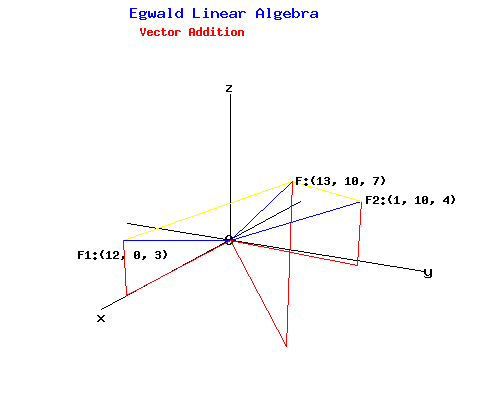Zero Vector.

The n dimensional vector o = (0, 0, . . . . ,0) is called the zero vector.

Properties under Scalar Multiplication and Vector Addition.

For scalars s1 and s2, and vectors u, v, and w in Rn:

 v + o = v v - v = o v + w = w + v (u + v) + w = u + (v + w) v + v = 2 * v 0 * v = o 1 * v = v -1 * v = -v s1 * (v + w) = s1 * v + s1 * w (s1 + s2) * v = s1 * v + s2 * v

The n-dimensional space Rn is a vector space, if its elements, vectors, obey the rules above.

Dot (Inner or Scalar) Product of two Vectors.

Given vectors v = [vi] and w = [wi] of dimension n, their dot product  v * w  is a scalar determined by:

v * w = v1 * w1 + v2 * w2 + . . . . + vn * wn,

ie the sum of the product of the vectors' components.

Length (Norm) of a vector.

Given a vector v = [vi] of dimension n, the length of v, denoted by ||v||2, is the square root of its own dot product:

||v||2 = sqrt(v * v) = sqrt(v12 + v22 + . . . . vn2),

||v||2 = (v * v)1/2

The ||.||2 norm is called the Euclidean norm of a vector. If v is a 2-dimensional vector, its formula is identical to the one used to find the hypotenuse of a right angled triangle.

If v = s, ie v is a 1-dimensional vector, then ||v||2 = |s|, the absolute value of the scalar s.

The p-norm of a vector.

The p-norm ||v||p of a vector v is:

||v||p = (|v1|p + |v2|p + . . . . |vn|p)1/p.

For p = 2 one gets the Euclidean norm.

For p = 1 one gets   ||v||p = (|v1| + |v2| + . . . . |vn|),   ie the sum of the absolute values of the vector's components.

For p = infinity one gets   ||v||inf = max1 <= i <= n {|vi|},   ie the component of the vector with the maximum absolute value.

Properties of Vector Norms.

For a given vector norm || . ||, any scalar s, and vectors v, w in Rn:

 ||v|| = 0 if and only if v = o, the zero vector. ||v|| > 0 for any non-zero vector v. ||s * v|| = |s| * ||v||. ||v + w|| <= ||v|| + ||w||.

The magnitude of a vector |v| = ||v||2, ie the length of v.

Geometric Interpretation of the Dot Product.

If ß is the angle from the vector v to the vector w, the dot product v * w is equivalent to:

v * w = |v| * |w| * cos(ß).

For vectors v = (12, 2) and w = (5, 9), ß = 0.9.

|v| * |w| * cos(ß) = 12.17 * 10.3 * cos(0.9) = 78

v * w = (12, 2) * (5, 9) = 78;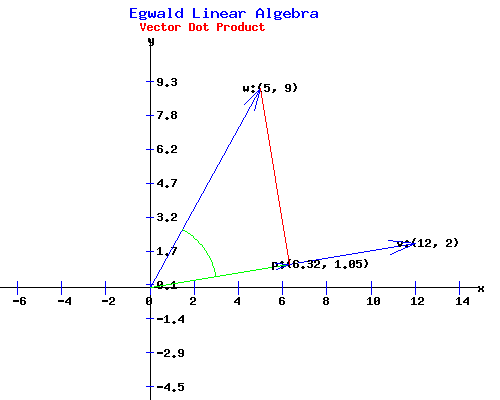From the diagram, one sees that |w| * cos(ß) is the length of the projection of w = (5, 9) onto v = (12, 2). Therefore, v * w is the length of vector p = (6.32, 1.05), times the length of v.

The vector p obtained by projecting w onto v can be obtained from the formula:

p = ( (v * w) / |v|) * (v / |v|) = ( (v * w) / |v|2) * v   =   (6.32, 1.05).

Scalar Product
v = [v1, v2] & w = [w1, w2]
v1: v2:
w1: w2:

You can change the vectors in the form above to see how v * w changes in the diagram. For example, try w = (5, -9) and click "Product".

Work equals Dot Product.

The work done by a constant force F = w, magnitude |w| = 10.3 newtons in the direction (5, 9), in displacing an object of mass 1 kg along the length 12.17 meters of the vector v = (12, 2) is v * w.

W = v * w = |w| newtons * |v| meters * cos(ß) = 78 newton meters (joules);

Properties of the Dot Product.

For any scalar s and vectors u, v, and w in Rn:

 (u + v) * w = u * v + u * w v * w = w * v (s * v) * w = s * (v * w) v * v >= 0 v * v = 0 if and only if v = o, the zero vector.

Euclidean Space.

The space Rn with the specified properties of scalar multiplication, vector addition, and inner product of vectors is called an Euclidean space of dimension n, denoted by En.

Vectors and Matrices.

Column and Row Vectors.

A vector v = (v1, v2, . . . . . , vn) in Rn can be specified as a column or row vector. By convention, v is an n by 1 array (a column vector), while its transpose   vT  is a 1 by n array (a row vector).

Matrices.

Given k vectors {v1, v2, . . . . vk} in Rn, where the components of the ith vector are given by:

vi = (v1, i, v2, i, . . . . vn, i)

the arrays

 v1, v2, . . . . vk = v1,1 v1,2     . . . . .    v1,k-1 v1,k v2,1 v2,2     . . . . .    v2,k-1 v2,k               . . . . . . . . . . .           vn,1 vn,2     . . .       vn,k-1 vn,k = V

are alternative ways of writing the matrix V. Thus V is an n by k array of scalars consisting of k n by 1 column vectors.

To understand the properties of matrices, and how matrices interact with vectors see the linear algebra: matrices web page.

Orthogonal Vectors.

Vectors v and w in Rn are said to be orthogonal if their inner product is zero. Since,

v * w = |v| * |w| * cos(ß),

where ß is the angle from v to w, non-zero vectors are orthogonal if and only if they are perpendicular to each other, ie when cos(ß) = 0.

Orthogonal vectors v and w are called orthonormal if they are of length one, ie v * v = 1, and w * w = 1.

Linear Dependence.

A set of k vectors {v1, v2, . . . . vk} in Rn is linearly dependent if a set of scalars {s1, s2, . . . . sk} can be found, not all zero, such that:

s1 * v1 + s2 * v2 + . . . . + sk * vk = 0.

If no such set of scalars exists, the k vectors {v1, v2, . . . . vk} are linearly independent.

Linear Combination.

A vector v depends linearly on vectors {v1, v2, . . . . vk} if scalars {s1, s2, . . . . sk} exist such that:

v = s1 * v1 + s2 * v2 + . . . . + sk * vk.

2-d Example.

The vector u = (14.25, 16.5) is a linear combination of the vectors v = (11, 4) and w = (4, 9) given by:

u = 0.75 * v + 1.5 * w = (14.25, 16.5).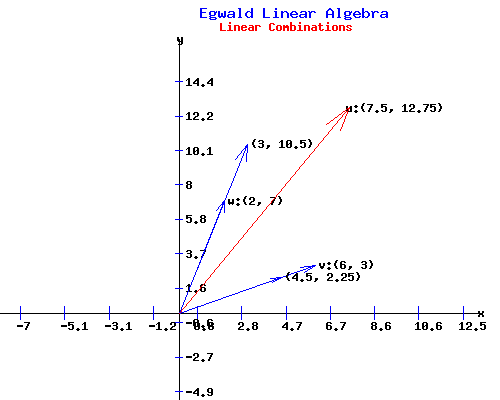Linear Combination
u = s1 * v + s2 * w
v1: v2:
w1: w2:
s1: s2:

You can change the vectors and the scalars in the form above to see how u = s1 * v + s2 * w changes in the diagram. For example, try s1 = 1.5 and s2 = .75 and click "Combination".

Vectors {v1, v2, . . . . vk} in Rn are linearly dependent if and only if one vector can be expressed as a linear combination of the remaining vectors.

The vector v depends linearly on the vector w if v = s * w for some scalar s.

The Span of a Set of Vectors.

Let V be a set of vectors {v1, v2, . . . . vk}. The span of the set of vectors in V, span(V), is the set of all linear combinations of the vectors in V:

span(V) = s1 * v1 + s2 * v2 + . . . . + sk * vk, for all possible sets of scalars {s1, s2, . . . . sk}.

Basis Vectors.

Unit Coordinate Vectors.

Write ei as the vector in Rn whose components are 0's except for the ith component which is a 1.

The vectors {e1, e2, . . . . en}, called the unit coordinate vectors, are orthonormal since the vectors satisfy ei * ei = 1, and ei * ej = 0 if i and j are different.

For example, in R3:

e1 = (1, 0, 0),

e2 = (0, 1, 0),

e3 = (0, 0, 1).

The vectors {e1, e2, . . . . en} in Rn are said to form a basis for Rn, since any vector v = (v1, v2, . . . . . , vn) in Rn can be expressed as a linear combination of the {e1, e2, . . . . en} vectors:

v = v1 * e1 + v2 * e2 + . . . . + vn * en,

ie the sum of the product of each component of v with the corresponding basis vector.

The linearly independent set of vectors {e1, e2, . . . . en} is said to span the n dimensional space Rn.

Other Basis Systems.

Theorem: Any basis of Rn consists of exactly n linearly independent vectors in Rn.

Theorem: Any n linearly independent vectors in Rn are a basis for Rn.

2-d Example.

Any two linearly independent vectors in R2 are a basis. Any three vectors in R2 are linearly dependent since any one of the three vectors can be expressed as a linear combination of the other two vectors.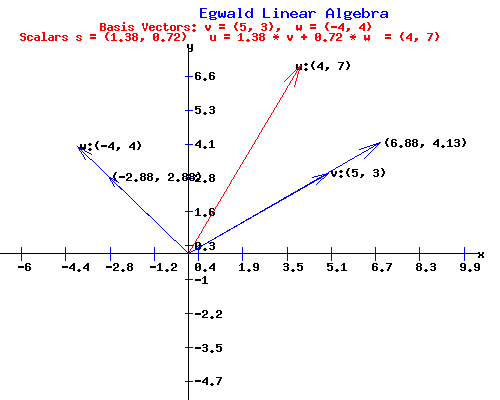Compute scalars s1 and s2 so that
u = s1 * v + s2 * w
v1: v2:
w1: w2:
u1: u2:

You can change the basis vectors and the vector u in the form above to see how the scalars s1 and s2 change in the diagram. For example, try u1 = -2 and u2 = 8 and click "Basis".

Change in Basis.

In the {e1, e1} basis, the vector u = u1 * e1 + u2 * e2 = 4 * e1 + 7 * e2.

In the {v, w} basis, the vector u = s1 * v + s2 * w = 1.38 * v + 0.72 * w.

To move from one basis to the other, write the matrix:

A = v,     w
 5 -4 3 4

Writing the coordinates u in the {v, w} basis as the vector s = (s1, s2)T, the product of the matrix A and the vector s equals u in the {e1, e1} basis:

u = A * s.

The matrix A has an inverse matrix,   A-1,   if and only if the vectors v and w are linearly independent. Then:

s = A-1 * u

s =
 0.125 0.125 -0.0938 0.1563
*
 4 7
=
 1.38 0.72

Linear Transformations

A linear transformation T from a n-dimensional space Rn to a m-dimensional space Rm is a function defined by a m by n matrix A such that:

y = T(x) = A * x,   for each x in Rn.

For example, the 2 by 2 change of basis matrix A in the 2-d example above generates a linear transformation from R2 to R2.

T(x) =
 5 -4 3 4
*
 x1 x2
=
 5 * x1 + -4 * x2 3 * x1 + 4 * x2
=
 y1 y2
= y

Transpose Linear Transformation.

The transpose transformation TT is a function from Rm to Rn obtained from the transpose of the matrix A used to define T:

TT(y) = AT * y.

Properties of Linear Transformations.

For any scalar s and vectors v and w in Rn, a linear transformation T from Rn to Rm obeys:

 T(v + w) = T(v) + T(w), T(s * v) = s * T(v).

Inverse Transformation T-1.

A linear transformation T has an inverse transformation T-1 if and only if the matrix A that defines T has an inverse matrix A-1.

If A-1 exists, then

x = T-1(y) = A-1 * y.

Note: T-1 = TT if and only if the matrix A used to define T is an orthogonal matrix.

The Image of a Transformation T.

Let A be an m by n matrix that defines a linear transformation T from Rn to Rm. Write A = {a1, a2, ... an} as the set of m by 1 column vectors of A, ie A = [a1, a2, ... an].

The image of T, im(T), is the span of the columns of A. Formally:

im(T) = span(A) = {y in Rm | y = A * x,  for some x in Rn.

Sometimes this is referred to as the image of the matrix A, im(A).

The Kernel of a Transformation T.

Define the linear transformation T(x) = A * x for A an m by n matrix. The kernel of T, ker(T), is the set of all vectors x in Rn for which T(x) = o, the zero vector in Rm.  Formally:

ker(T) = {x in Rn | A * x = 0  in Rm}

Sometimes this is referred to as the kernel of the matrix A, ker(A).

3-d Example.

Define the linear transformation T from R3 to R3 by T(x) = A * x where:

T(x) = A * x =
 12 1 0 8 10 0 1 3 0
*
 x1 x2 x3

The image of T, im(T), is the plane (2 dimensional) in R3 spanned by the vectors of the first two columns of A as displayed in the following diagram.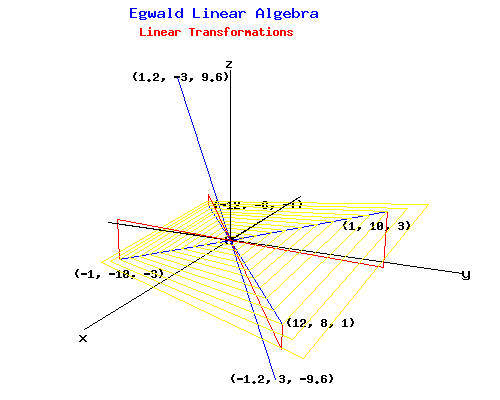The kernel of T, ker(T), coincides with the z-axis, since the product of A and any vector xT = (0, 0, x3) equals o in R3.

The transpose linear transformation TT from R3 to R3 is defined by AT as:

TT(y) = AT * y =
 12 8 1 1 10 3 0 0 0
*
 y1 y2 y3

The im(TT) coincides with the x-y plane in R3, orthogonal to ker(T).

The ker(TT) coincides with the line through the vector yT = (1.2, -3, 9.6), orthogonal to im(T).

Subspaces of Rn.

Let V be a subset of the set of all vectors in Rn.   V is said to be a vector subspace of Rn if V has the following properties:

 V contains the zero vector o, If v and w are in V, then v + w is in V, If s is a scalar and v is in V, then s * v is in V.

Examples of Vector Subspaces.

a. If T is a linear transformation from Rn to Rm, then im(T) and ker(TT) are vector subspaces of Rm, while ker(T) and im(TT) are vector subspaces of Rn.

b. If V is any set of k vectors {v1, v2, . . . . vk} in Rn, then span(V) is a vector subspace of Rn.

Gram-Schmidt Process.

Given a set of k linearly independent vectors {v1, v2, . . . . vk} that span a vector subspace V of Rn, the Gram-Schmidt process generates a set of k orthogonal vectors {q1, q2, . . . . qk} that are a basis for V.

The Gram-Schmidt process is based on an idea contained in the following diagram.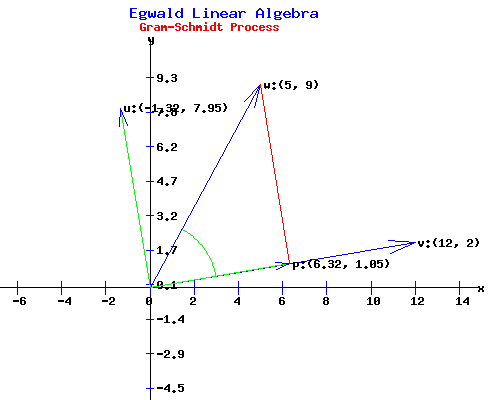From the diagram above, the vector p obtained by projecting of w = (5, 9) onto v = (12, 2) is p = (6.32, 1.05).

The projection vector is obtained by:

p = ( (v * w) / (v * v) ) * v = 0.527 * v = (6.32, 1.05).

The vector u perpendicular to v is obtained by subtracting the projection of w onto v from w leaving that part of w that is perpendicular to v as the vector u:

u = w - p = (5, 9) - (6.32, 1.05) = (-1.32, 7.95).

Gram-Schmidt Process
v = [v1, v2] & w = [w1, w2]
v1: v2:
w1: w2:

You can change the vectors in the form above. For example, try w = (5, -9) and click "Gram-Schmidt".

3-d Example.

Use the Gram-Schmidt process to obtain an orthogonal basis for the two vectors that span the im(T) subspace.

v, w
 12 1 8 10 1 3

The projection of w on v is the vector p given by:

p = ((v * w) / (v * v)) * v = 0.4545 * v

Subtracting p from w yields u, a vector perpendicular to v.

u =
 1 10 3
- 0.4545 *
 12 8 1
=
 -4.4545 6.3636 2.5455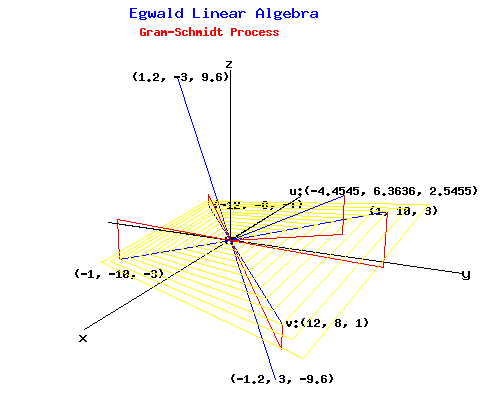The vectors v and u are an orthogonal basis for im(T). Dividing these two vectors by their norms yields an orthonormal basis for the vector subspace.

Orthonormal Basis.

It is desirable to obtain an orthonormal basis for a vector subspace because it is easy to work with an orthonormal basis. On the matrices web page, the Gram-Schmidt process is used to construct an orthonormal basis from a set of linearly independent vectors.

Specifically, the orthonormal basis matrix Q was obtained for R4:

Q =
 q1 q2 q3 q4
 0.2132 0.6617 -0.6199 0.3638 0.4264 0.0389 -0.31 -0.8489 0.2132 0.6617 0.7085 -0.1213 0.8528 -0.3503 0.1328 0.3638

Orthonormal Basis Representation of a Vector.

Since the set {q1, q2, q3, q4} is a basis for R4, any vector bT = (b1, b2, b3, b4) in R4 can be written as a linear combination of the {qk} basis vectors.

b = s1*q1 + s2*q2 + s3*q3 + s4*q4.

To obtain each scalar sk, notice that qi * qj = 0 if i and j are different, while qk * qk = 1. Therefore, multiplying the expression for b by each basis vector qkT one obtains each scalar multiplier sk:

qkT * b  =  sk.

Substituting these values for sk into the expression for b:

b = (qT1 * b)*q1 + (qT2 * b)*q2 + (qT3 * b)*q3 + (qT4 * b)*q4.

Example.

Let the vector bT = ( 1, 1, 1, 1). The vector of scalar multipliers, s = ( 1.7056, 1.012, -0.0886, -0.2426). In terms of the orthonormal basis vectors:

b = (1.7056) * q1 + (1.012) * q2 + (-0.0886) * q3 + (-0.2426) * q4

Vector Cross Product.

While the vector dot product of two vectors produces a scalar, the vector cross product combines two vectors to produce a third vector perpendicular to the first two vectors. Using the conventions of Analytic Geometry, define the unit coordinate vectors in R3 by

i = (1, 0, 0),

j = (0, 1, 0),

k = (0, 0, 1).

Let v = v1i + v2j + v3k  and  w = w1i + w2j + w3k  be two vectors in R3. Their cross product v X w is the vector:

u = v X w = (v2 w3 - v3 w2)i + (v3 w1 - v1 w3)j + (v1 w2 - v2 w1)k.

3-d Example.

Change the values for the vectors v and w in the form below and click "Cross Product" to see how their cross product,  u  changes.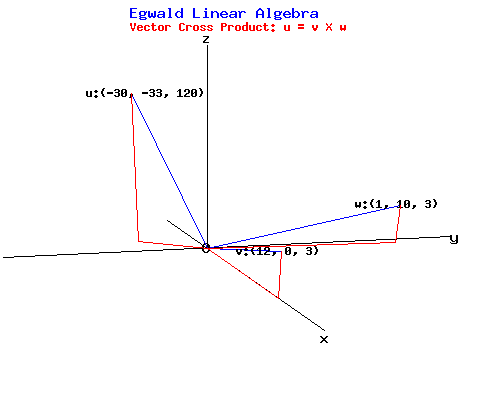Compute Vector Cross Product
u = v X w
v1: v2: v3:
w1: w2: w2:

Given vectors:

v = 12i + 3j + 7k,   w = 1i + 10j + 3k,

their vector cross product is:

u = v X w = (3 * 3 - 7 * 10)i + (7 * 1 - 12 * 3)j + (12 * 10 - 3 * 1)k = -61i + -29j + 117k.

References.

• Apostle, Tom M. Calculus, Vol. 1. New York: Blaisdell, 1962.
• Ayres, Frank Jr. Matrices. New York: Schaum McGraw-Hill, 1962.
• Ayres, Frank Jr. Modern Algebra. New York: Schaum McGraw-Hill 1965.
• Bretscher, Otto. Linear Algebra with Applications. Upper Saddle River: Prentice Hall, 1997.
• Cohn, P. M. Linear Equations. London: Routledge, 1964.
• Cohn, P. M. Solid Geometry. London: Routledge, 1961.
• Cutnell, John D. and Kenneth W. Johnson. Physics. 3rd ed. New York: John Wiley, 1995.
• Dowling, Edward T. Mathematics for Economists. New York: Schaum McGraw-Hill, 1980.
• Lipschutz, Seymour. Linear Algebra. New York: Schaum McGraw-Hill, 1968.
• Spiegel, Murray R. Vector Analysis and an Introduction to Tensor Analysis. New York: Schaum, 1959.
• Thomas, George B. Calculus and Analytical Geometry. Reading, Mass.: Addison-Wesley, 1960.Copyright © Elmer G. Wiens:   EgwaldWeb ServicesAll Rights Reserved.    Inquiries